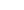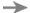# Analytical Method

The Analytical Method is a generic process combining the power of the Scientific Method with the use of formal process to solve any type of problem. It has these nine steps:

1. Identify the problem to solve.

2. Choose an appropriate process. (THE KEY STEP)3. Use the process to hypothesize analysis or solution elements.4. Design an experiment(s) to test the hypothesis.5. Perform the experiment(s).6. Accept, reject, or modify the hypothesis.7. Repeat steps 3, 4, 5, and 6 until the hypothesis is accepted.

8. Implement the solution.

9. Continuously improve the process as opportunities arise.

## Why this is important

Use of the Analytical Method is critical to solving the sustainability problem because it appears that current processes are inadequate. They are intuitive, simple, and based on how activists approach everyday problems.

## Application example

The key step in the Analytical Method is selecting an appropriate process. Suppose you are starting out on solving a tough problem. That's the first step. You have identified the problem to solve.

The next step is to choose an appropriate process. Depending on the problem it should have varous amounts of these features. At a minimum, the process should:

1. Decompose the problem into the right subproblems.

2. Find the root causes of each subproblem.

3. Find the high leverage points for resolving each root cause.

4. Find the solutions for pushing on the high leverage points.

For an example of how these four steps have been performed on the sustainability problem, please see the Common Property Rights book.

## Elaboration

Let's derive the Analytical Method from scratch. We start with the problem solivng process all of us use for everyday problems. It's a simple, quickly performed procedure that works just fine for easy problems. It's performed so fast it's intuitive and we seldom think about it. Its main steps are:

The Intuitive Method

1. Identify the problem to solve.

2. Quickly intuitively find the solution. (THE WEAK STEP)

3. Implement the solution.

What's missing? Analysis. There's no analysis step, causing step two to be weak.

The Intuitive Method is the norm in environmentalism. Environmentalists do analyze. But what they are analyzing is not the problem as a whole. It's tiny pieces of the problem, like R&D for new forms of alternative energy or what's the best way to reduce a certain form of pollution.

These are superficial solutions. They attempt to solve intermediate causes of the problem. Superficial solutions are intuitively derived from a review of an intermediate cause of interest. For example, too much burning of fossil fuel is seen as a problem to solve. Its solution is switching to forms of alternative energy like solar and wind power. But what is the deeper cause of too much burning of fossil fuel? Why isn't the system already self-correcting, since we now know burning of fossil fuel causes climate change? Analysis questions like these are needed, so we can find the root causes. Fixing the root causes will ripple up the causal chain and fix the intermediate causes the root causes were causing.

So let's add an analysis step:

A Vastly Better Method

1. Identify the problem to solve.

2. Perform an analysis. (KEY STEP)

3. Develop a solution based on the analysis.

4. Implement the solution.

This raises a question: How do we perform a high quality analysis? The only way science and business have found to do that reliably is by using a process that defines how the analysis should be done. So let's add a select-a-process step:

An Almost Good Enough Method

1. Identify the problem to solve.

2. Choose an appropriate process to perform the analysis. (KEY STEP)

3. Execute the process. Its output is a high quality analysis.

4. Develop a solution based on the analysis.

5. Implement the solution.

This raises a further question: How do we choose an appropriate process?

That question was answered definitively by the invention of the Scientific Method by Galileo and Francis Bacon in the 14th and 15th centuries. The Scientific Method is the great granddaddy of all modern problem solving processes. It has these five amazingly simple but powerful steps:

The Scientific Method

1. Observe a phenomenon that has no good explanation.

2. Formulate a hypothesis.

3. Design an experiment(s) to test the hypothesis.

4. Perform the experiment(s).

5. Accept, reject, or modify the hypothesis.

So we don't have to choose a process at the highest appropriate level of abstraction. We already have one: the Scientific Method. Let's incorporate it into the five steps we had earlier:

The Analytical Method

1. Identify the problem to solve.

2. Choose an appropriate process. (THE KEY STEP)3. Use the process to hypothesize analysis or solution elements.4. Design an experiment(s) to test the hypothesis.5. Perform the experiment(s).6. Accept, reject, or modify the hypothesis.7. Repeat steps 3, 4, 5, and 6 until the hypothesis is accepted.

8. Implement the solution.

9. Continuously improve the process as opportunities arise.

We've also added the last step of continuous process improvement. This is mandatory until you've solved the same type of problem many times. Then your process will have matured.

This gives us the nine steps of the Analytical Method. All scientifically based problem solving approaches use the Analytical Method. By listing its steps we can see exactly where we need to improve to perform it well. The crux is usually step 2, choose an appropriate process to use the Scientific Method to analyze and develop a solution to the problem.

For that we have developed the System Improvement Process. It's the process that drives all the work at Thwink.org.

We hope that someday a suitable process drives your work too.

Browse the GlossaryPreviousNextRELATED INFORMATION
The Greatest Invention in the Last 10,000 Years

The Scientific Method is arguably the most significant invention since the invention of agriculture. Before it was invented, Homo sapiens had no reliable way to test new knowledge. Unsound and sound principles mixed freely because no one could tell the difference. That's why bleeding was widely used in medicine. It's why science was called alchemy. It's why so called scientists searched for the "philosopher's stone," which could turn base materials into gold.

But once the Scientific Method was invented, everything changed. The invention of new reliable knowledge accelerated by several orders of magnitude. The result was the Scientific Revolution, which was followed by the Industrial Revolution, which ultimately created the page you are reading now.

Analytical Methods JournalUse of a sufficiently powerful Analytical Method is so crucial to scientific problem solving that in March 2009 a new journal was announced: Analytical Methods. This is "A peer-reviewed journal highlighting the advancement of analytical technologies for wider application by the international scientific community."

Despite the wording, here "analytical method" means composition analysis of compounds of interest, such as food, archelogical artifacts, forensic work, the medical industry, and so on. But the concept has much broader application.